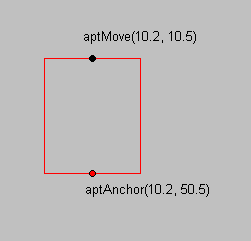﻿ L_AnnDefine2 | Function References | Raster Imaging C API Help
In This Topic ▼

# L_AnnDefine2

#include "l_bitmap.h"

L_LTANN_API L_INT L_AnnDefine2(hObject, apt, uState)

Specifies the shape when creating or transforming an annotation object. This function specifies one point in the current window each time it is called.

## Parameters

### HANNOBJECT hObject

Handle to the annotation object.

### pANNPOINT apt

Pointer to an ANNPOINT structure. The specified point is in container coordinates.

### L_UINT uState

State of the process of modifying the annotation object. Possible values are:

Value Meaning
ANNDEFINE_SETANCHORPOINT  This is an anchor point. Use this value when rotating an annotation object around a point other than its center. When doing this, call L_AnnDefine2 with this flag set prior to calling L_AnnDefine2 with the ANNDEFINE_BEGINROTATE flag set.
ANNDEFINE_BEGINMOVEPOINT  This is a starting point, used when moving one point on an annotation object. This can be used to move a single point on any of the following annotation objects:
ANNOBJECT_LINE
ANNOBJECT_POLYLINE
ANNOBJECT_POLYGON
ANNOBJECT_POINTER
ANNOBJECT_FREEHAND
ANNOBJECT_FREEHANDHOTSPOT
ANNOBJECT_RULER
ANNOBJECT_CROSSPRODUCT
ANNOBJECT_PROTRACTOR
ANNOBJECT_CURVE
ANNOBJECT_CURVECLOSED
You can use ANNDEFINE_BEGINMOVEPOINT to perform a simultaneous rotate and resize for the following objects:
(Note that you must set an anchor point prior to using ANNDEFINE_BEGINMOVEPOINT)
ANNOBJECT_RECT
ANNOBJECT_ELLIPSE
ANNOBJECT_HILITE
ANNOBJECT_REDACT
ANNOBJECT_TEXT
ANNOBJECT_NOTE
ANNOBJECT_STAMP
ANNOBJECT_HOTSPOT
When doing this, call L_AnnDefine2 with the ANNDEFINE_SETANCHORPOINT flag set prior to calling L_AnnDefine2 with the ANNDEFINE_BEGINMOVEPOINT flag set.

#### Returns

Value Meaning
SUCCESS The function was successful.
< 1 An error occurred. Refer to Return Codes.

This function is used only with the following states:

• ANNDEFINE_SETANCHORPOINT
• ANNDEFINE_BEGINMOVEPOINT

If you try to use a `uState` value other than ANNDEFINE_SETANCHORPOINT or ANNDEFINE_BEGINMOVEPOINT, the function returns ERROR_INV_PARAMETER.

This function is similar to L_AnnDefine, except that L_AnnDefine2 takes a point in container coordinates, whereas L_AnnDefine takes a point in client coordinates.

Use this function when additional accuracy is required when setting an anchor point, or doing a simultaneous rotate and resize. This function should be used in conjunction with L_AnnDefine.

As an example, suppose you want to rotate and resize the rectangle around the anchor point aptAnchor by moving the point aptMove, as shown in the following figure:This can be done by using only L_AnnDefine, but because L_AnnDefine takes integral arguments and aptAnchor and aptMove are not integral, the rotate and resize will not be exact.

To solve this and accurately perform simultaneous rotation and resizing, use L_AnnDefine2 and L_AnnDefine as follows:

````ANNPOINT aptAnchor;    `
`ANNPOINT aptMove;   `
`aptMove.x = 10.2;   `
`aptMove.y = 10.5   `
`aptAnchor.x = 10.2;   `
`aptAnchor.y = 50.5   `
`ptLocation.x = 10;   `
`ptLocation.y = 20;   `
`L_AnnDefine2(hObject,  &aptAnchor, ANNDEFINE_SETANCHORPOINT);   `
`L_AnnDefine2(hObject,  &aptMove, ANNDEFINE_BEGINROTATE);   `
`// One or more ANNDEFINE_APPEND   `
`L_AnnDefine(pData->hObjectChange, &pMousePos->pt, ANNDEFINE_APPEND);    `
`L_AnnDefine(pData->hObjectChange, &pMousePos->pt, ANNDEFINE_END);  ````

Required DLLs and Libraries

Platforms

Win32, x64.

## Example

This example uses static variables to simulate user input to rotate/resize a rectangle around a point on the bottom center line of the rectangle.
Initially the rectangle must not be rotated.

````L_INT AnnDefine2Example(HANNOBJECT hRect) `
`{ `
`   L_INT nRet; `
`   // Counter for simulating user input `
`   static L_INT   nCallCount = 0; `
`   L_UINT         uType; `
`   ANNRECT        arcRect; `
`   ANNPOINT       aptAnchor;  // Anchor point in container coordinates `
`   ANNPOINT       aptMove;    // Move point in container coordinates `
`   POINT          ptMove;     // Move point in client coordinates `
`   L_DOUBLE       dAngle; `
`   HANNOBJECT     hContainer; `
` `
`   nRet = L_AnnGetType(hRect, &uType); `
`   if(nRet != SUCCESS) `
`      return nRet; `
`   if (uType != ANNOBJECT_RECT) `
`   { `
`      MessageBox(NULL, TEXT("Object must be a rectangle."), TEXT("Error"), MB_OK); `
`      return 0; `
`   } `
` `
`   nRet = L_AnnGetRotateAngle(hRect, &dAngle); `
`   if(nRet != SUCCESS) `
`      return nRet; `
`   if (dAngle != 0) `
`   { `
`      MessageBox(NULL, TEXT("Object must not be rotated."), TEXT("Error"), MB_OK); `
`      return nRet; `
`   } `
`   ++nCallCount; `
`   switch (nCallCount) `
`   { `
`   case 1: `
`      nRet = L_AnnGetRect(hRect, &arcRect, NULL); `
`      if(nRet != SUCCESS) `
`         return nRet; `
`      aptAnchor.x = (arcRect.left + arcRect.right) / 2; `
`      aptAnchor.y = arcRect.bottom; `
` `
`      aptMove.x = (arcRect.left + arcRect.right) / 2; `
`      aptMove.y = arcRect.top; `
` `
`      nRet = L_AnnDefine2(hRect, &aptAnchor, ANNDEFINE_SETANCHORPOINT); `
`      if(nRet != SUCCESS) `
`         return nRet; `
`      nRet = L_AnnDefine2(hRect, &aptMove, ANNDEFINE_BEGINMOVEPOINT); `
`      if(nRet != SUCCESS) `
`         return nRet; `
`      MessageBox(NULL, TEXT("Call ExampleAnnDefine2 to complete the rotate/resize."), TEXT(""), MB_OK); `
`      break; `
` `
`   case 2: `
`      nRet = L_AnnGetRect(hRect, &arcRect, NULL); `
`      if(nRet != SUCCESS) `
`         return nRet; `
`      aptMove.x = arcRect.right; `
`      aptMove.y = arcRect.top; `
` `
`      nRet = L_AnnGetTopContainer(hRect, &hContainer); `
`      if(nRet != SUCCESS) `
`         return nRet; `
`      nRet = L_AnnConvert(hContainer, &ptMove, &aptMove, 1, ANNCONVERT_TO_CLIENT); `
`      if(nRet != SUCCESS) `
`         return nRet; `
` `
`      nRet = L_AnnDefine(hRect, &ptMove, ANNDEFINE_END); `
`      if(nRet != SUCCESS) `
`         return nRet; `
`      nCallCount = 0; `
`      MessageBox(NULL, TEXT("Rotate/resize finished!"), TEXT(""), MB_OK); `
`      break; `
`   } `
`   return SUCCESS; `
`} ````

Help Version 20.0.2020.4.3